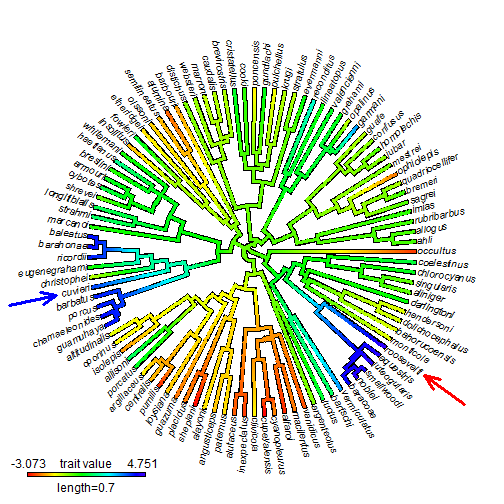## Sunday, December 14, 2014

I just wrote a function to automatically add an arrow to a radial (`"fan"`) tree plot. This could be adapted to trees of different types of course. The purpose of it is to add arrows that point to specific tips or nodes. For aesthetic reasons, we'd like the angle of the arrow to match the angle of the terminal edge of the tree leading to our target leaf.

Here is what my code looks like:

``````add.arrow<-function(tree=NULL,tip,...){
lastPP<-get("last_plot.phylo",envir=.PlotPhyloEnv)
if(is.numeric(tip)){
ii<-tip
if(!is.null(tree)&&ii<=length(tree\$tip.label)) tip<-tree\$tip.label[ii]
else tip<-""
} else if(is.character(tip)&&!is.null(tree)) ii<-which(tree\$tip.label==tip)
strw<-lastPP\$cex*(strwidth(tip)+strwidth("W"))
if(hasArg(arrl)) arrl<-list(...)\$arrl
else {
if(lastPP\$type=="fan") arrl<-0.3*max(lastPP\$xx)
else if(lastPP\$type=="phylogram") arrl<-0.15*max(lastPP\$xx)
}
if(hasArg(hedl)) hedl<-list(...)\$hedl
else hedl<-arrl/3
if(hasArg(angle)) angle<-list(...)\$angle
else angle<-45
arra<-angle*pi/180
if(hasArg(col)) col<-list(...)\$col
else col<-"black"
if(hasArg(lwd)) lwd<-list(...)\$lwd
else lwd<-2
if(lastPP\$type=="fan"){
theta<-atan2(lastPP\$yy[ii],lastPP\$xx[ii])
segments(x0=lastPP\$xx[ii]+cos(theta)*(strw+arrl),
y0=lastPP\$yy[ii]+sin(theta)*(strw+arrl),
x1=lastPP\$xx[ii]+cos(theta)*strw,
y1=lastPP\$yy[ii]+sin(theta)*strw,
col=col,lwd=lwd,lend="round")
segments(x0=lastPP\$xx[ii]+cos(theta)*strw+cos(theta+arra/2)*hedl,
y0=lastPP\$yy[ii]+sin(theta)*strw+sin(theta+arra/2)*hedl,
x1=lastPP\$xx[ii]+cos(theta)*strw,
y1=lastPP\$yy[ii]+sin(theta)*strw,
col=col,lwd=lwd,lend="round")
segments(x0=lastPP\$xx[ii]+cos(theta)*strw+cos(theta-arra/2)*hedl,
y0=lastPP\$yy[ii]+sin(theta)*strw+sin(theta-arra/2)*hedl,
x1=lastPP\$xx[ii]+cos(theta)*strw,
y1=lastPP\$yy[ii]+sin(theta)*strw,
col=col,lwd=lwd,lend="round")
}
}
``````

Note that I use three calls to `segments` rather than more obvious graphics function `arrows`. This is because I was unhappy with the options for user control of the dimensions of the arrow head in `arrows`, so I ensentially built my own version.

And now I'll use it to add arrows to an anole tree plotted in the `"contMap"` style.

``````library(phytools)
obj
``````
``````## Object of class "contMap" containing:
##
## (1) A phylogenetic tree with 101 tips and 100 internal nodes.
##
## (2) A mapped continuous trait on the range (-3.072659, 4.751288).
``````
``````plot(obj,type="fan",lwd=3,fsize=c(0.8,1),legend=0.7,
outline=TRUE)This is for a paper in which we use `locate.yeti` to place the little known & possibly extinct taxon Anolis roosevelti into the Caribbean Anolis phylogeny. Note that the ML placement (sister to the clade containing A. equestris is not where we a priori would've expected it to belong, that is, as sister to A. cuvieri; however nor can we statistically reject that placement. For more on that, see our paper - which is in revision, but we hope will ultimately be accepted and come out next year.